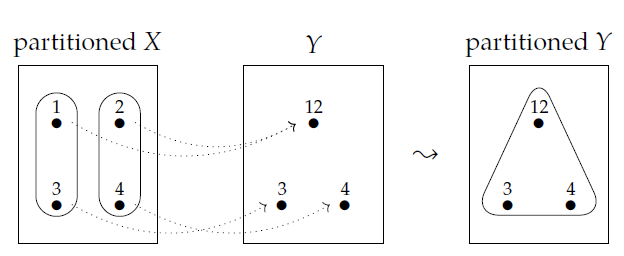I've spent four lectures on the logic of partitions; you may be wondering why. One reason was to give you examples illustrating this important fact: **Theorem.** Left adjoints preserve joins and right adjoints preserve meets. Suppose \$$f : A \to B\$$ and \$$g : B \to A\$$ are monotone functions between posets. Suppose that \$$f\$$ is the left adjoint of \$$g\$$, or equivalently, \$$g\$$ is the right adjoint of \$$f\$$. If the join of \$$a,a' \in A\$$ exists then so does the join of \$$f(a), f(a') \in B\$$, and $f(a \vee a') = f(a) \vee f(a').$ If the meet of \$$b,b' \in B\$$ exists then so does the meet of \$$g(b), g(b') \in A\$$, and $g(b \wedge b') = g(b) \wedge g(b').$ The proof is very easy, so this deserves being called a "Theorem" only because it's so fundamental! I will prove it later, in more generality. Right now let's see how it's relevant to what we've been doing. In [Lecture 9](https://forum.azimuthproject.org/discussion/1931/lecture-9-chapter-1-adjoints-and-the-logic-of-subsets/p1) we saw something interesting about the subsets. Given any set \$$X\$$ there's a poset \$$P(X)\$$ consisting of all subsets of \$$X\$$. Given any function \$$f : X \to Y\$$ there's a monotone map $f^* : P(Y) \to P(X)$ sending any subset of \$$Y\$$ to its preimage under \$$f\$$. And we saw that \$$f^{\ast} \$$ has both a left adjoint and a right adjoint. This means that \$$f^{\ast} \$$ _is_ both a right adjoint and a left adjoint. (Remember: _having_ a left adjoint means _being_ a right adjoint, and vice versa.) So by our Theorem, we see that \$$f^* : P(Y) \to P(X)\$$ preserves both meets and joins! You can also see this directly - see Puzzle 41 in [Lecture 13](https://forum.azimuthproject.org/discussion/2008/lecture-13-chapter-1-pulling-back-partitions/p1). But what matters here is the general pattern. In [Lecture 13](https://forum.azimuthproject.org/discussion/2008/lecture-13-chapter-1-pulling-back-partitions/p1) we also saw something interesting about partitions. Given any set \$$X\$$ there's a poset \$$\mathcal{E}(X)\$$ consisting of all partitions of \$$X\$$. Given any function \$$f : X \to Y\$$ there's a monotone map $f^* : \mathcal{E}(Y) \to \mathcal{E}(X)$ sending any partition of \$$Y\$$ to its pullback along \$$f\$$. And we saw that while \$$f^{\ast} \$$ preserves meets, it does not preserve joins! So by our Theorem, we see that \$$f^* : \mathcal{E}(Y) \to \mathcal{E}(X)\$$ _cannot_ be a left adjoint. On the other hand, it _might_ be a right adjoint. And indeed it is! So, this strange difference between the logic of subsets and the logic of partitions is really all about adjoints. **Puzzle 42.** Given a function \$$f : X \to Y\$$ there is a way to **push forward** any partition \$$P\$$ on \$$X\$$ and get a partition \$$f_{!} (P)\$$ on \$$Y\$$. In pictures it looks like this:although this is not the most exciting example, since here \$$f_{!}(P)\$$ has just one part. Figure out the precise general description of \$$f_{!} (P)\$$. If you get stuck read Section 1.5.2 of _[Seven Sketches](http://math.mit.edu/~dspivak/teaching/sp18/7Sketches.pdf)_. **Puzzle 43.** Show that for any function \$$f : X \to Y\$$, pushing forward partitions along \$$f\$$ gives a monotone map $f_{!} : \mathcal{E}(X) \to \mathcal{E}(Y) .$ **Puzzle 44.** Show that for any function \$$f : X \to Y\$$, \$$f^* : \mathcal{E}(Y) \to \mathcal{E}(X)\$$ is the right adjoint of \$$f_{!}: \mathcal{E}(X) \to \mathcal{E}(Y)\$$. **[To read other lectures go here.](http://www.azimuthproject.org/azimuth/show/Applied+Category+Theory#Course)**